## Saturday, 9 August 2014

### CHAPTER 7 - Worked Out Examples

 Example: 7
Find the sum$S$ given by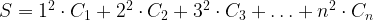$S = {1^2} \cdot {C_1} + {2^2} \cdot {C_2} + {3^2} \cdot {C_3} + \ldots + {n^2} \cdot {C_n}$
 Solution: 7
We have to plan an approach wherein we are able to generate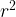${r^2}$ with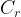${C_r}$. We can generate one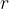$r$ with every${C_r}$, as we did earlier, and which is now repeated here: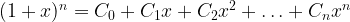${(1 + x)^n} = {C_0} + {C_1}x + {C_2}{x^2} + \ldots + {C_n}{x^n}$
Differentiating both sides with respect to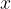$x$, we have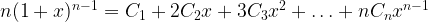$n{(1 + x)^{n - 1}} = {C_1} + 2{C_2}x + 3{C_3}{x^2} + \ldots + n{C_n}{x^{n - 1}}$
Now we have reached the stage where we have an$r$ with every${C_r}$. We need to think how to get the other$r$. If we differentiate once again, we’ll have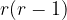$r(r - 1)$ with every${C_r}$ instead of${r^2}$ (understand this point carefully). To ‘make-up’ for the power that falls one short of the required value, we simply multiply by$x$ on both sides of the relation above to obtain: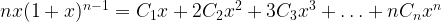$nx{(1 + x)^{n - 1}} = {C_1}x + 2{C_2}{x^2} + 3{C_3}{x^3} + \ldots + n{C_n}{x^n}$
It should be evident now that the next step is differentiation: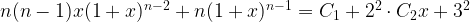$n(n - 1)x{(1 + x)^{n - 2}} + n{(1 + x)^{n - 1}} = {C_1} + {2^2} \cdot {C_2}x + {3^2}$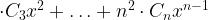$\cdot {C_3}{x^2} +\ldots + {n^2} \cdot {C_n}{x^{n - 1}}$
Now we simply substitute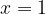$x = 1$ to obtain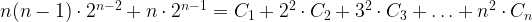$n(n - 1) \cdot {2^{n - 2}} + n \cdot {2^{n - 1}} = {C_1} + {2^2} \cdot {C_2} + {3^2} \cdot {C_3} +\ldots + {n^2} \cdot {C_n}$
The required sum$S$ is thus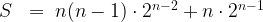$S\;\; = \;n(n - 1) \cdot {2^{n - 2}} + n \cdot {2^{n - 1}}$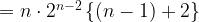$= n \cdot {2^{n - 2}}\left\{ {(n - 1) + 2} \right\}$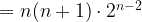$= n(n + 1) \cdot {2^{n - 2}}$
 Example: 8
Evaluate the following sums:
 (a)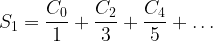${S_1} = \dfrac{{{C_0}}}{1} + \dfrac{{{C_2}}}{3} + \dfrac{{{C_4}}}{5} +\ldots$ (b)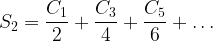${S_2} = \dfrac{{{C_1}}}{2} + \dfrac{{{C_3}}}{4} + \dfrac{{{C_5}}}{6} +\ldots$
 Solution: 8-(a)
The first sum contains only the even-numbered binomial coefficients, while the second contains only odd-numbered ones. Recall that we have already evaluated the sum$S$ given by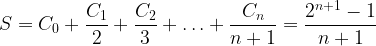$S = {C_0} + \dfrac{{{C_1}}}{2} + \dfrac{{{C_2}}}{3} + \ldots + \dfrac{{{C_n}}}{{n + 1}} = \dfrac{{{2^{n + 1}} - 1}}{{n + 1}}$
Note that$S$ is the sum of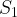${S_1}$ and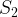${S_2}$, i.e.,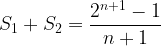${S_1} + {S_2} = \dfrac{{{2^{n + 1}} - 1}}{{n + 1}}$
Thus, if we determine${S_1}$ ,${S_2}$ is automatically determined, and vice-versa. Let us try to determine${S_1}$ first.
Consider again the general expansion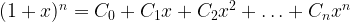${(1 + x)^n} = {C_0} + {C_1}x + {C_2}{x^2} +\ldots + {C_n}{x^n}$
Integrating with respect to$x$, we have (we have not yet decided the limits)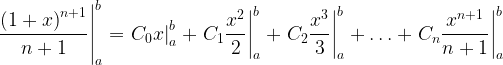$\left. {\dfrac{{{{(1 + x)}^{n + 1}}}}{{n + 1}}} \right|_a^b = \left. {{C_0}x} \right|_a^b + \left. {{C_1}\dfrac{{{x^2}}}{2}} \right|_a^b + \left. {{C_2}\dfrac{{{x^3}}}{3}} \right|_a^b +\ldots + \left. {{C_n}\dfrac{{{x^{n + 1}}}}{{n + 1}}} \right|_a^b$
Since we are trying to determine${S_1}$ which contains only the even-numbered terms, we have to choose the limits of integration such that the odd-numbered terms vanish. This is easily achievable by setting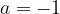$a = - 1$ and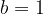$b = 1$ (understand this carefully). Thus, we have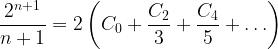$\dfrac{{{2^{n + 1}}}}{{n + 1}} = 2\left( {{C_0} + \dfrac{{{C_2}}}{3} + \dfrac{{{C_4}}}{5} + \ldots } \right)$
which implies that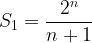${S_1} = \dfrac{{{2^n}}}{{n + 1}}$
 Solution: 8-(b)${S_2}$ is now simply given by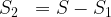${S_2}\;\; = S - {S_1}$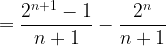$= \dfrac{{{2^{n + 1}} - 1}}{{n + 1}} - \dfrac{{{2^n}}}{{n + 1}}$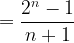$= \dfrac{{{2^n} - 1}}{{n + 1}}$